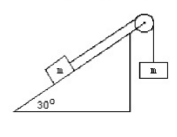Problem: In the figure below, two masses, each 150 kg, are NOT at rest and are attached to each other by thin unstretchable cable. Find the acceleration of this system of masses and cable. The angle of the ramp is as shown in the figure. (Hint: Draw a free-body diagram for each mass) Find the magnitude and direction of the acceleration of the system if the coefficient of friction between block and ramp is 0.

FREE Expert Solution
97% (266 ratings)
Problem Details

In the figure below, two masses, each 150 kg, are NOT at rest and are attached to each other by thin unstretchable cable. Find the acceleration of this system of masses and cable. The angle of the ramp is as shown in the figure. (Hint: Draw a free-body diagram for each mass) Find the magnitude and direction of the acceleration of the system if the coefficient of friction between block and ramp is 0.